# Class 10 Light Reflection and Refraction Previous Years Questions

Light Reflection and Refraction Previous Years Questions

1. The image distance from the eye lens in the normal eye when we increase the distance of an object from the eye
1. increases
2. decreases
3. remains unchanged
4. depends on the size of the eyeball [1 mark] [CBSE 2020]
2. Four students A, B, C and D carried out measurement of focal length of a concave mirror as shown in the following four diagrams1. A 2. B 3. C 4. D [1 mark] [CBSE 2017]
3. If you have to determine the focal length of a convex lens, You should have:
1. a convex lens and a screen.
2. a convex lens and a lens holder.
3. a lens holder, a screen holder and a scale.
4. a convex lens, a screen, holders for them and a scale. [1 mark] [CBSE 2017]
4. To determine the approximate value of the focal length of a given concave mirror, you focus the image of a distant object formed by the mirror on a screen. The image obtained on the screen, as compared to the object is always :
1. Laterally inverted and diminished
2. Inverted and diminished
3. Erect and diminished
4. Erect and highly diminished [1 mark] [CBSE 2017]
5. In your laboratory you trace the path of light rays through a glass slab for different values of angle of incidence (∠i) and in each case measure the values of the corresponding angle of refraction (∠r) and angle of emergence (∠e). On the basis of your observations your correct conclusion is:
1. ∠i is more than ∠r, but nearly equal to ∠e
2. ∠i is less than ∠r, but nearly equal to ∠e
3. ∠i is more than ∠e, but nearly equal to ∠r
4. ∠i is less than ∠e, but nearly equal to ∠r [1 mark] [CBSE 2017]
6. In an experiment on tracing the path of a ray of light through a rectangular glass slab, four students A,B,C and D used the following values of angle of incidence and the distance between the two pins. (fixed on the line representing the incident ray):
1. (30 degree, 45 degree, 60 degree) and 2 cm
2. (30 degree, 45 degree, 60 degree) and 8 cm
3. (20 degree, 50 degree, 70 degree) and 2 cm
4. (20 degree, 50 degree, 70 degree) and 8 cm
The student has taken the correct values is:
1. (1)
2. (2)
3. (3)
4. (4) [1 mark] [CBSE 2017]
7. A student while doing experiment could not place the prism accurately on the table. The right way to place the prism in front of him while doing experiment is: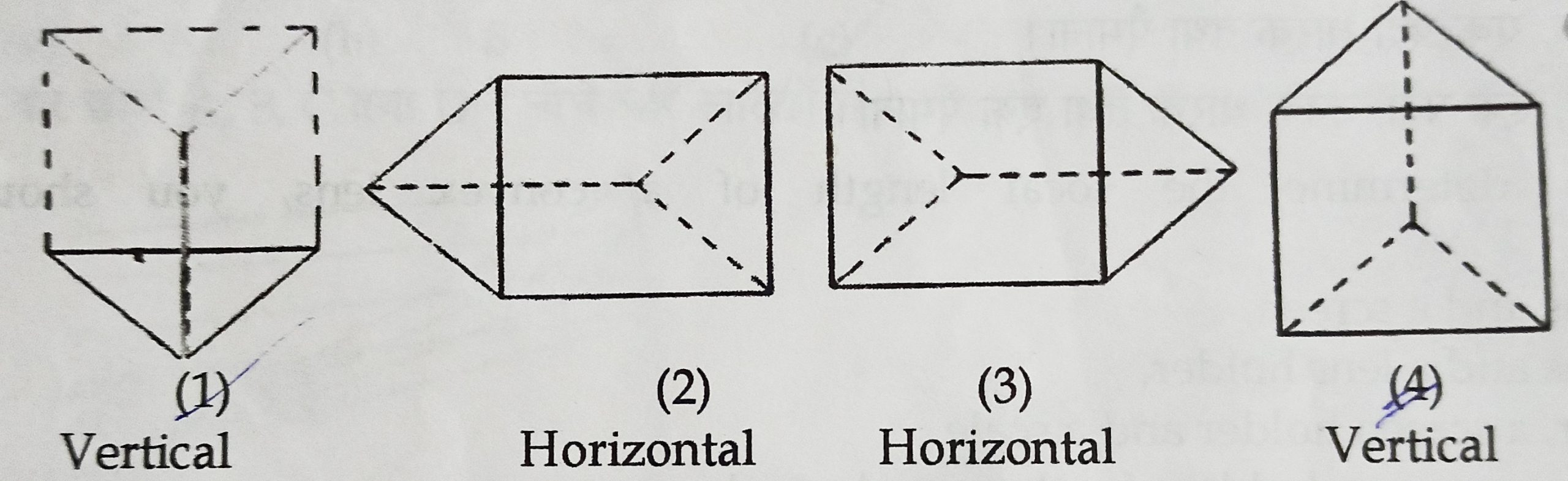1. fig. (1) and (2)
2. fig. (2) and (3)
3. fig. (3) and (4)
4. fig. (4) [1 mark] [CBSE 2017]
8. In the following ray diagram the correctly marked angles are:1. ∠i and ∠e
2. ∠A and ∠D
3. ∠i, ∠e and ∠D
4. ∠r, ∠A and ∠D [1 mark] [CBSE 2016]
9. Suppose you have focused on a screen the image of candle flame placed at the farthest end of the laboratory table using a convex lens. If your teacher suggests you to focus the parallel rays of the sun, reaching your laboratory table, on the same screen, what you are expected to do is to move the :
1. lens slightly towards the screen
2. lens slightly away from the screen
3. lens slightly towards the sun
4. lens and screen both towards the sun. [1 mark] [CBSE 2016]
10. A student focused the sun rays using an optical device ‘X’ on a screen S as shown.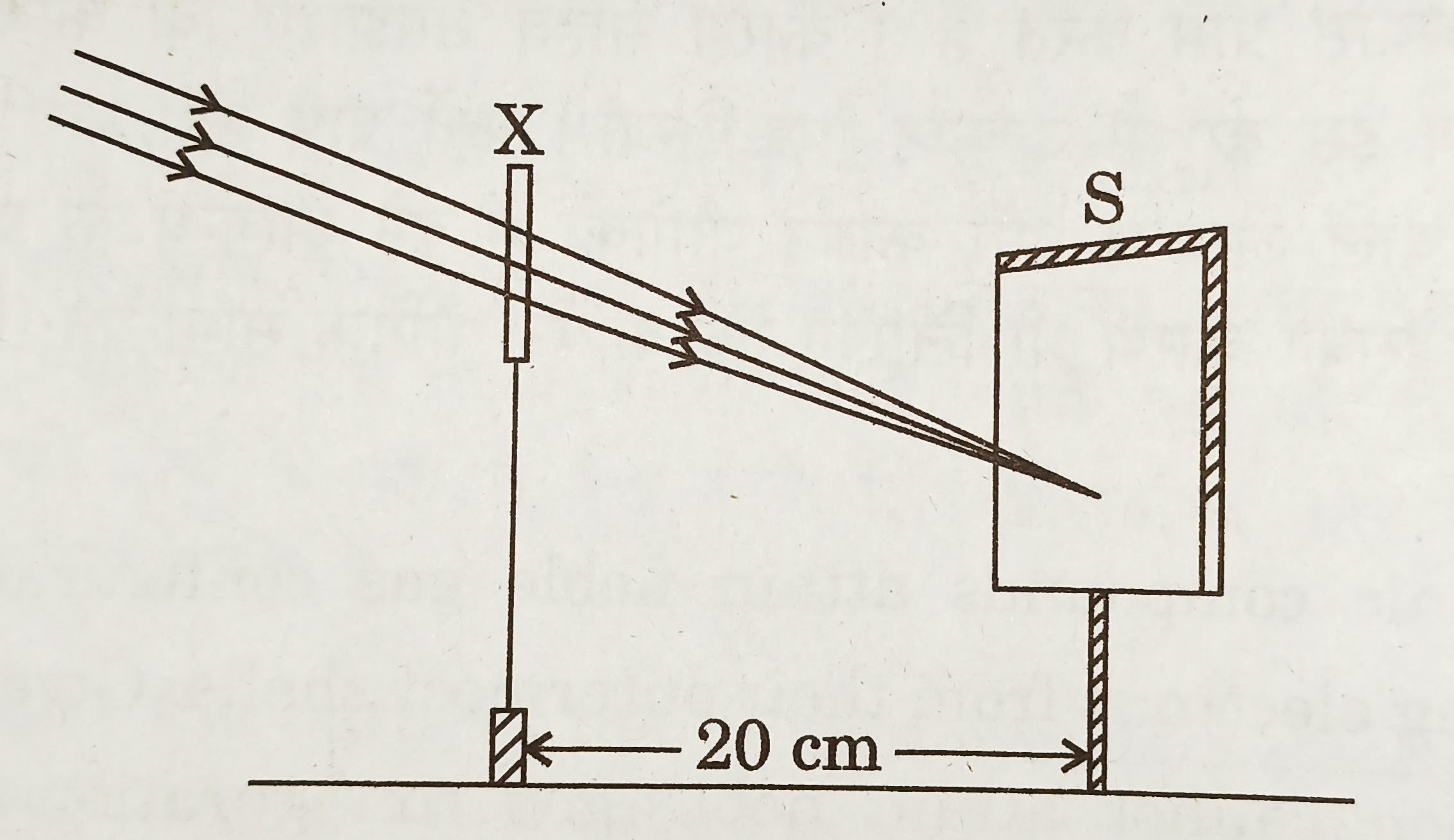From this it may be concluded that the device ‘X’ is a (select the correct option)
1. Convex lens of focal length 10 cm.
2. Convex lens of radius of curvature 20 cm.
3. Convex lens of focal length 20 cm.
4. Convex mirror of focal length 20 cm. [1 mark] [CBSE 2014]
11. A student has obtained an image of a well-illuminated distant object on a screen to determine the focal length, F1 of the given spherical mirror. The teacher then gave him another mirror of focal length, F2 and asked him to obtain a focused image of the same object on the same screen. The student found that in order to focus the same object using the second mirror, he has to move the mirror away from the screen. From this observation it may be concluded that both the spherical mirrors given to the student were (select the correct option)
1. Concave and F1 < F2
2. Concave and F1 > F2
3. Convex and F1 < F2
4. Convex and F1 > F2 [1 mark] [CBSE 2014]
12. A student is using a convex lens of focal length 18 cm to study the image formation by it for the various positions of the object. He observes that when he places the object at 27 cm, the location of the image is at 54 cm on the other side of the lens. Identify from the following diagram the three rays that are obeying the laws of the refraction and may be used to draw the corresponding ray diagram .1. 1, 2 and 4
2. 1, 3 and 5
3. 2, 4 and 5
4. 2, 3 and 4 [1 mark] [CBSE 2014]
13. A student is using a convex lens of focal length 10 cm to study the image formation by a convex lens for the various positions of the object. In one of his observations, he may observe that when the object is placed at a distance of 20 cm from the lens, its image is formed at (select the correct option)
1. 20 cm on the other side of the lens and is of the same size, real and erect.
2. 40 cm on the other side of the lens and is magnified, real and inverted.
3. 20 cm on the other side of the lens and is of the same size, real and inverted.
4. 20 cm on the other side of the lens and is of the same size, virtual and erect. [1 mark] [CBSE 2014]
14. In the following diagram, the path of a ray of light passing through a glass prism is shown: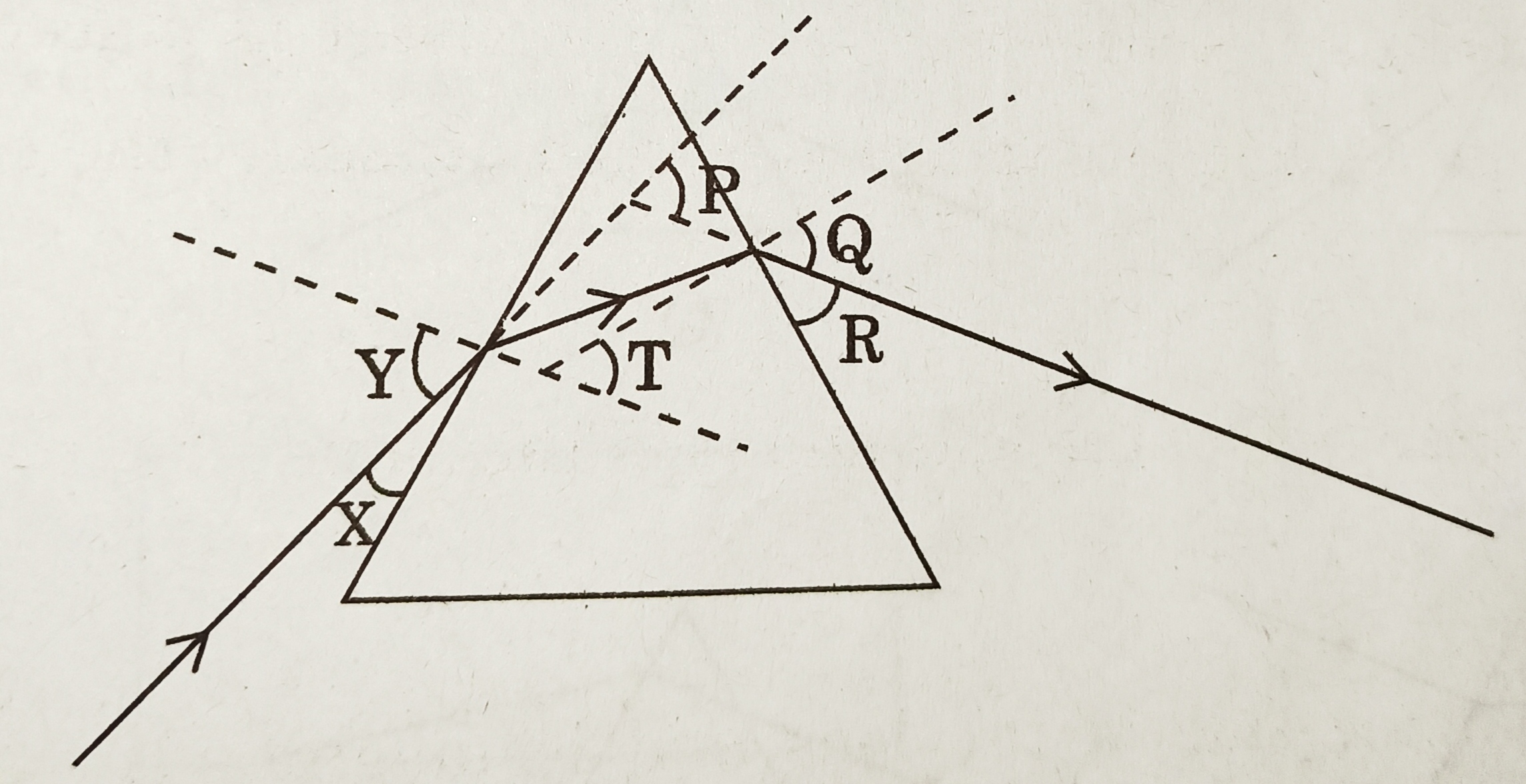In this diagram the angle of incidence, the angle of emergence and the angle of deviation respectively are (select the correct option)
1. X, R and T
2. Y, Q and T
3. X, Q and P
4. Y, Q and P [1 mark] [CBSE 2014]
15. Study the following diagrams in which the path of a ray of light passing through a glass prism as traced by four students P, Q, R and S is shown:The student who has traced the path correctly is
1. P
2. Q
3. R
4. S [1 mark] [CBSE 2014]
16. A student traces the path of a ray of light passing through a rectangular glass slab and marks the angle of incidence i, angle of refraction r and angle of emergence e, as shown.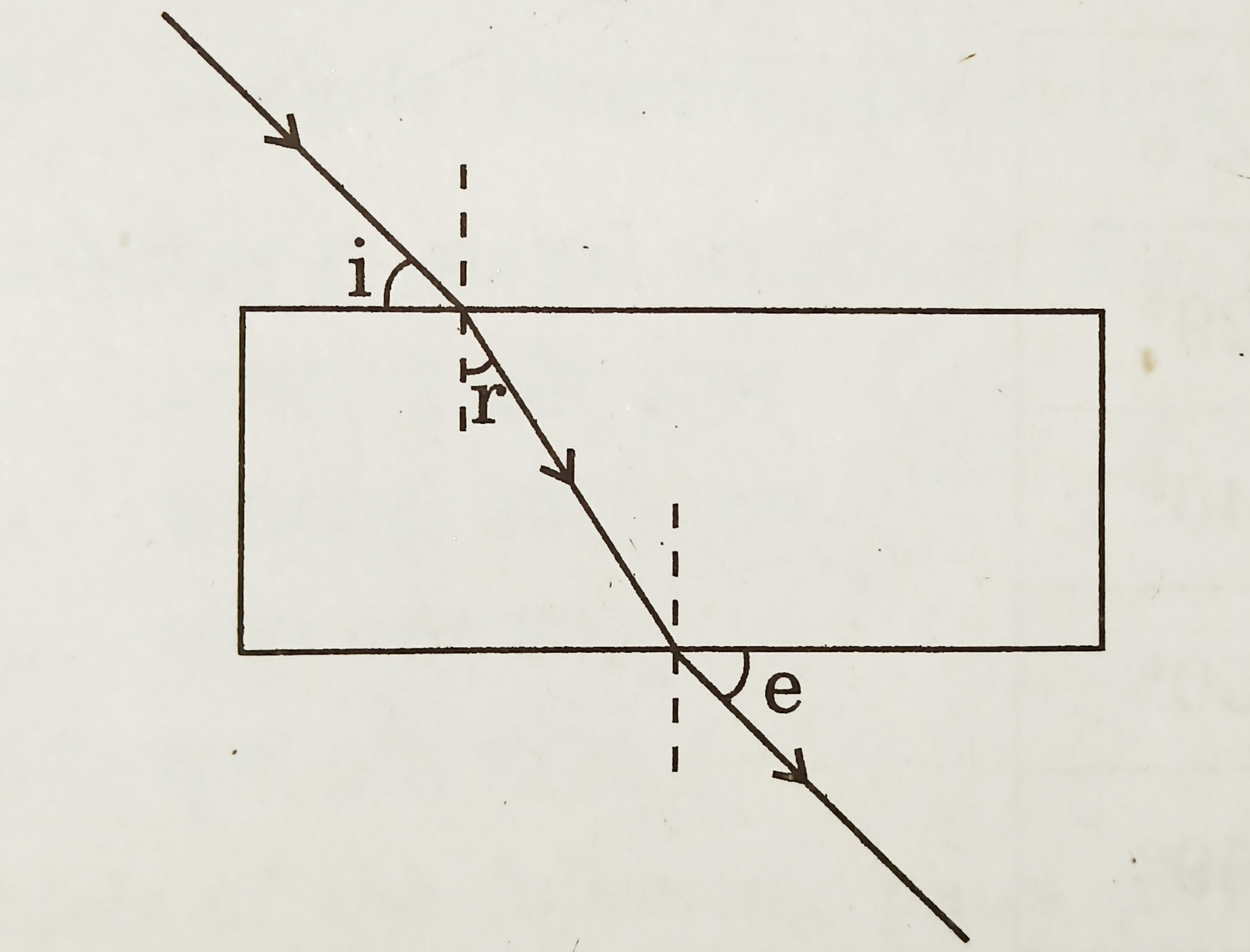The correctly marked angle (s) is/are
1. ∠i only
2. ∠e only
3. ∠r only
4. ∠i and ∠e [1 mark] [CBSE 2014]
17. In the ray diagram, AB is an object placed in front of a convex lens L1L2. F1 and F2 are its foci. F1OF2 is principal axis.Complete the ray diagram to locate the position of image formed after refraction through it. Also compare the size of the object and the image. [2 marks] [CBSE 2017]
18. List in proper sequence the steps of the experiment for determining the approximate focal length of a given concave mirror by obtaining the image of a distant object. [2 marks] [CBSE 2019]
19. A student has to trace the path of a ray of light passing through a rectangular glass slab for four different values of angle of incidence.
1. Write two important precautions for this experiment.
2. List two conclusions the student will draw based on his experiment. [2 marks] [CBSE 2019]
20. If the image formed by a spherical mirror for all positions of the object placed in front of it is always erect and diminished, what type of mirror is it? Draw a labelled ray diagram. [2 marks] [CBSE 2018]
21. An object of height 4.0 cm is placed at a distance of 30 cm from the optical centre ‘O’ of a convex lens of focal length 20 cm. Draw a ray diagram to find the position and size of the image formed. Mark optical centre ‘O’ and principal focus ‘F’ on the diagram. Also find the approximate ratio of size of the image to the size of the object. [2 marks] [CBSE 2018]
22. “The magnification produced by a spherical mirror is -3”. List four informations you obtain from this statement about the mirror image. [2 marks] [CBSE 2016]
23. An object of height 2.5 cm is placed at a distance of 15 cm from the optical centre ‘O’ of a convex lens of focal length 10 cm. Draw a ray diagram to find the position and size of the image formed. Mark optical centre ‘O’, principal focus F and height of the image on the diagram. [2 marks] [CBSE 2017]
24. The image formed by a concave mirror is observed to be virtual, erect and larger than the object. Where should the position of the object be relative to the mirror? Draw ray diagram to justify your answer. [2 marks] [CBSE 2014]
25. Draw a ray diagram to show the path of the refracted ray in each of the following case:
A ray of light incident on a concave lens is
1. passing through its optical centre.
2. parallel to its principal axis.
3. directed towards its principal focus. [3 marks] [CBSE 2014]
26. A students wants to project the image of a candle flame on a screen 48 cm in front of a mirror by keeping the flame at a distance of 12 cm from its pole.
1. Suggest the type of mirror he should use.
2. Find the linear magnification of the image produced.
3. How far is the image from the object?
4. Draw the ray diagram to show the image formation in this case. [3 marks] [CBSE 2014]
27. A narrow beam PQ of white light is passing through a glass prism ABC as shown in the diagram.Trace it on your answer sheet and show the path of the emergent beam as observed on the screen DE.
1. Write the name and cause of the phenomena observed.
2. Where else in nature is this phenomena observed?
3. Based on this observation, state the conclusion which can be drawn about the constituents of white light. [3 marks] [CBSE 2014]
28. The image of an object formed by a lens is of magnification -1. If the distance between the object and its image is 60 cm, what is the focal length of the lens ? If the object is moved 20 cm towards the lens, where would the image be formed ? State reason and also draw a ray diagram in support of your answer. [3 marks] [CBSE 2016]
29. Describe an activity to show that the colours of white light splitted by a glass prism can be recombined to get white light by another identical glass prism. Also draw ray diagram to show the recombination of spectrum of white light. [3 marks] [CBSE 2016]
30. State the laws of refraction of light. Explain the term ‘absolute refractive index of a medium’and write an expression to relate it with the speed of light in vaccum. [3 marks] [CBSE 2018]
31. What is meant by power of lens? Write its S.I. unit. A student uses a lens of focal length 40 cm and another of -20 cm. Write the nature and power of each lens. [3 marks] [CBSE 2018]
32. Draw ray diagram in each of the following cases to show what happens after reflection to the incident ray when
1. it is parallel to the principal axis & falling on a convex mirror.
2. it is falling on a concave mirror while passing through its principal focus.
3. it is coming oblique to the principal axis and falling on the pole of a convex mirror. [3 Marks] [CBSE 2020]
33. One half of a convex lens is covered with a black paper.
1. Show the formation of image of an object placed at 2F1, of such covered lens with the help of ray diagram. Mention the position and nature of image.
2. Draw the ray diagram for same object at same position in front of the same lens, but now uncovered. Will there be any difference in the image obtained in the two cases? Give reason for your answer. [3 Marks] [CBSE 2017]
34. .
1. List four characteristics of the image formed by a concave lens of focal length 20 cm when the object is placed at a distance of 40 cm from its optical centre.
2. The size of image of an object by a convex lens of focal length 20 cm is observed to be reduced to 1/3rd of its size. Find the distance of the object from the optical centre of the lens. [5 marks] [CBSE 2019]
35. .
1. List four characteristics of the images formed by plane mirrors.
2. A 5 cm tall object is placed at a distance of 20 cm from a concave mirror of focal length 30 cm. Use mirror formula to determine the position and size of the image formed. [5 marks] [CBSE 2019]
36. .
1. Draw a labelled ray diagram to show the path of a ray of light incident obliquely on one face of a glass slab.
2. Calculate the refractive index of the material of a glass slab. Given that the speed of light through the glass slab is 2 x 108 m/s and in air is 3 x 108 m/s.
3. Calculate the focal length of a lens, if its power is -2.5 D. [5 marks] [CBSE 2020]
37. .
1. In the figure given below mark the angle of prism, angle of deviation for red and violet colour and the incident ray.
2. Explain how the components of white light can be recombined after a prism has separated them. Explain with the help of figure.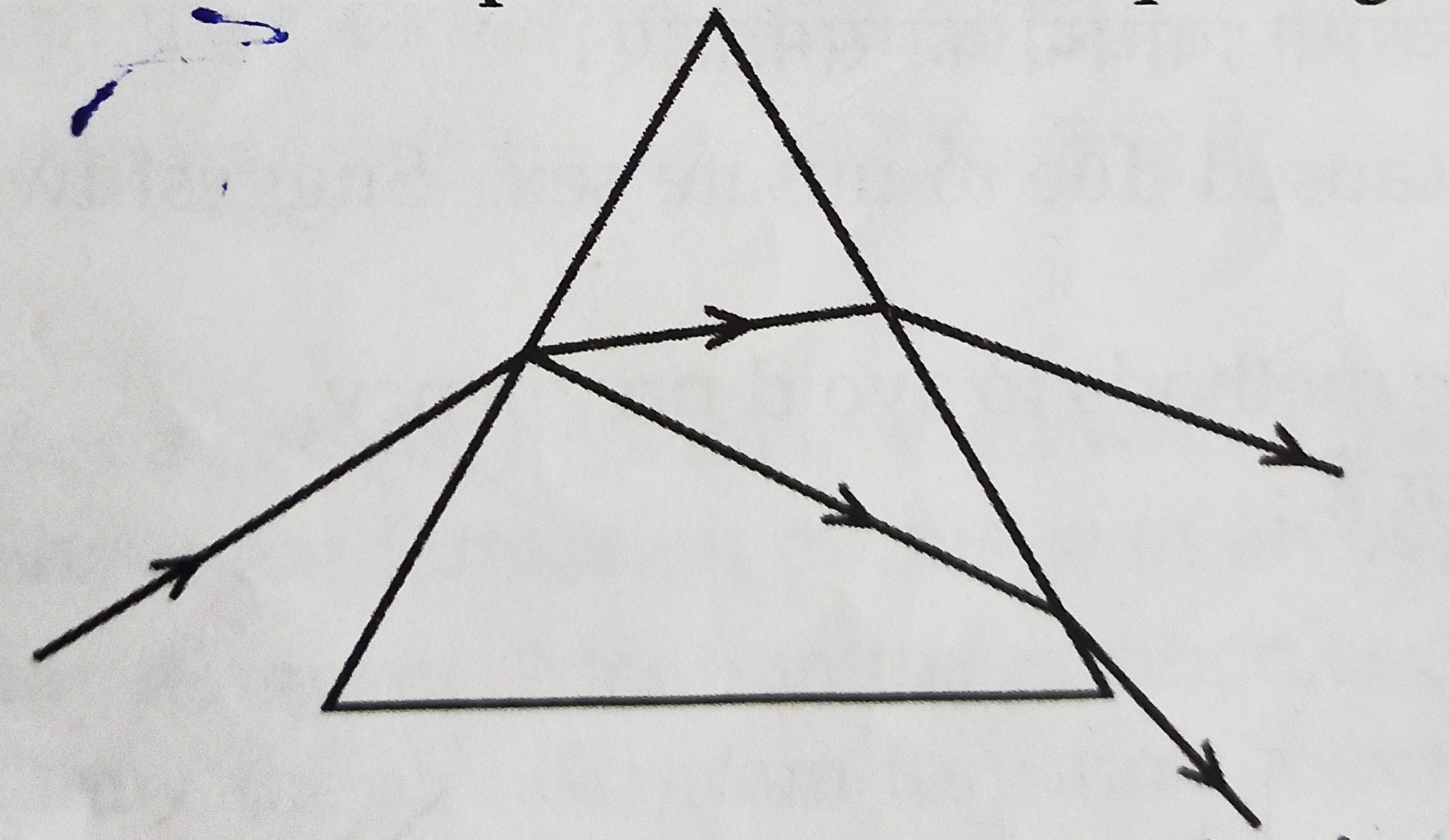[5 marks] [CBSE 2017]
38. An object is placed at 20 cm from a concave mirror of focal length 10 cm. Find the position and nature of image formed. Draw ray diagram to explain the formation of image.(not to scale). [5 marks] [CBSE 2020]
39. .
1. Complete the following ray diagram: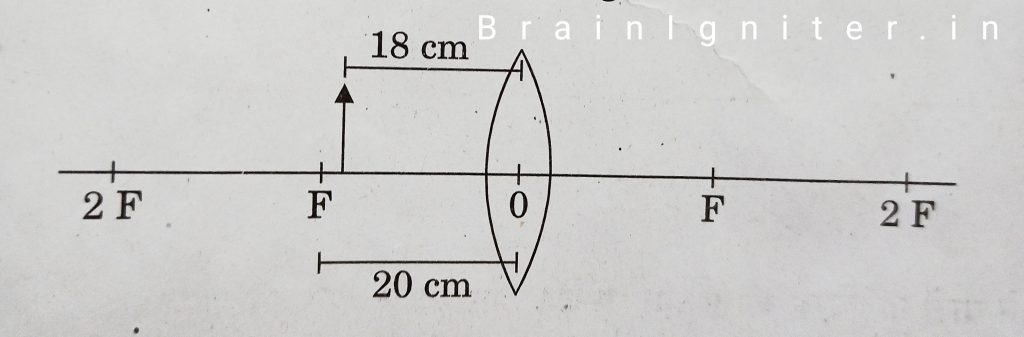2. Find the nature, position and size of the image formed.
3. Use lens formula to determine the magnification in this case. [5 marks] [CBSE 2020]
40. .
1. Complete the following ray diagram:2. Find the nature, position and size of the image formed.
3. Use mirror formula to determine the magnification in this case. [5 marks] [CBSE 2020]
41. It is desired to obtain an erect image of an object, using concave mirror of focal length of 12 cm.
1. What should be the range of distance of an object placed in front of the mirror?
2. Will the image be smaller or larger than the object. Draw ray diagram to show the formation of image in this case.
3. Where will the image of this object be, if it is placed 24 cm in front of the mirror ? Draw ray diagram for this situation also to justify your answer.
Show the positions of pole, principal focus and the centre of curvature in the above ray diagrams. [5 marks] [CBSE 2016]
42. .
1. Define focal length of a spherical lens.
2. A divergent lens has a focal length of 30 cm. At what distance should an object of height 5 cm from the optical centre of the lens be placed so that its image is formed 15 cm away from the lens? Find the size of the image also.
3. Draw a ray diagram to show the formation of image in the above situation. [5 marks] [CBSE 2016]
43. Explain the following terms related to spherical lenses:
1. Centres of curvature
2. Principal axis
3. Optical centre
4. Principal focus
At what distance from a concave lens of focal length 20 cm, should a 6 cm tall object be placed so that it forms an image at 15 cm from the lens ? Also determine the size of the image formed. [5 marks] [CBSE 2014]

43. Refractive indices of four media A, B, C and D are given below:

(a) In which of the given media is the speed of the light maximum and minimum ?
(b) Which of them is the most optically dense and which one is the least optically dense medium ?
(c) Calculate refractive index of medium B with respect to medium C. Give reasons for your answers. [5 marks] [CBSE 2017]

44. After tracing the path of a ray of light passing through a rectangular glass slab for four different values of the angle of incidence, a student reported his observations in tabular form as given below:

The best observations is
(A) I (B) II (C) III (D) IV [1 mark] [CBSE 2014]